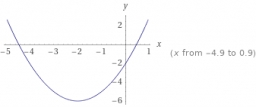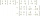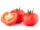# Missing digit

If 4x/6x + 5/17 = 1, what digit does the x represents

x =  8

### Step-by-step explanation:Did you find an error or inaccuracy? Feel free to write us. Thank you!Tips to related online calculators
Need help to calculate sum, simplify or multiply fractions? Try our fraction calculator.
Do you have a linear equation or system of equations and looking for its solution? Or do you have a quadratic equation?

## Related math problems and questions:

• 5 2/55 2/5 hours a week  mathematics,  3 3/4 hours a week   Natural sciences, 4 3/8 hours a week  Technology . how many hours does  he spend on social sciences if he spend 17 1/2 hours a week for the four subject?
• Multiplicative inverseExplain why the reciprocal of - 1 1/17 is the multiplicative inverse of - 17/18.
• Meanif the mean of the set of data 5, 17, 19, 14, 15, 17, 7, 11, 16, 19, 5, 5, 10, 8, 13, 14, 4, 2, 17, 11, x is -91.74, what is the value of x?
• Equation with fractionsWhat is the simplified form of the equation fraction 4 over 5 n minus fraction 1 over 5 equals fraction 2 over 5 n? n = -2 n = 4 n = fraction 1 over 2
• Equation with fractionsSolve an equation: 1- (2x-5)/(6) =3- (x)/(4) It is an equation with fractions.
• If-then equationIf 1/2 + 2/5s = s - 3/4 what is the value of s?
• Fractions and mixed numerals(a) Convert the following mixed numbers to improper fractions. i. 3 5/8 ii. 7 7/6 (b) Convert the following improper fraction to mixed number. i. 13/4 ii. 78/5 (c) Simplify these fractions to their lowest terms. i. 36/42 ii. 27/45 2. evaluate following ex
• Four-digit numbersHow many four-digit numbers can we make from the numbers 2, 6, 3, 5, 1 and 9 if the numbers in the number cannot be repeated?
• Fraction of a NumberIf 1/2 of 1/3 of 1/4 of 1/5 of a number is 2.5. what is the number?
• FractionsThree-quarters of an unknown number is 4/5. What is 5/6 of this unknown number?
• To improper fractionChange mixed number to improper fraction a) 1 2/15 b) -2 15/17
• EquationSolve equation and check the result: 1.4x - 3/2 + x - 9,8 = x + 0,4/3 - 7 + 1,6/6
• Missing numberBlank +1/6 =3/2 find the missing number
• Evaluate expressionEvaluate expression using BODMAS rule: 1 1/4+1 1/5÷3/5-5/8
• Unknown number 6Determine x if 1/6 of x is equal to 2/5 of the number 24.
• Over fractionSolve equation: p over 5 equals fraction numerator 7 p over denominator 6 end fraction plus 5
• The ketchupIf 3 1/4 of tomatoes are needed to make 1 bottle of ketchup. Find the number of tomatoes required to make 4 1/5 bottles